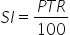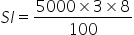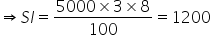Maths-
General
Easy

Question

# Find the simple interest and amount on Rs 5000 in 3 years at 8% p.a.Hint:

## The correct answer is: 6200 Rupees

### Complete step by step solution:We calculate simple interest by the formula,…(i)where P is Principal amount, T is number of years and R is rate of interestHere, we have T = 3,R = 8% and P = 5000On substituting the known values in (i), we getWe have SI = 1200 Rupees.We know the formula for total amount = A = P + SI…(ii)where A is the total amount, P is the principal amount and SI is simple interest.On substituting the known values in (ii), we get A = 5000 + 1200 = 6200Hence total amount to be paid after 3 years = A = 6200 Rupees.#### With Turito Foundation.#### Get an Expert Advice From Turito.